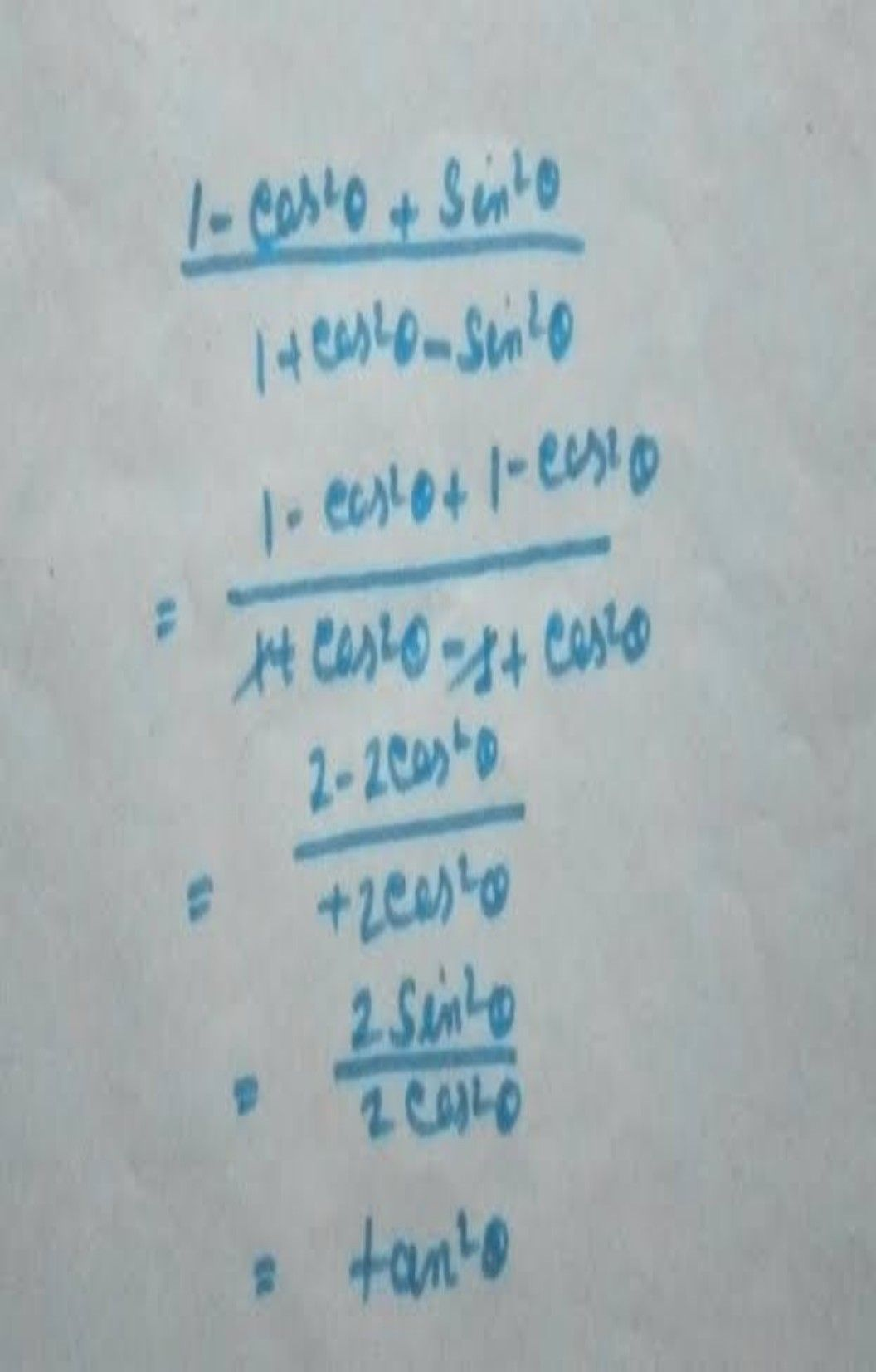Symbol
Problem$1-$ $cos2$ theta $+sin2$ theta $\div 1+cos2$ theta $sin2$ theta $=ta$ $1an$ theta
Trigonometry
Search count: 118
SolutionQanda teacher - priyanka